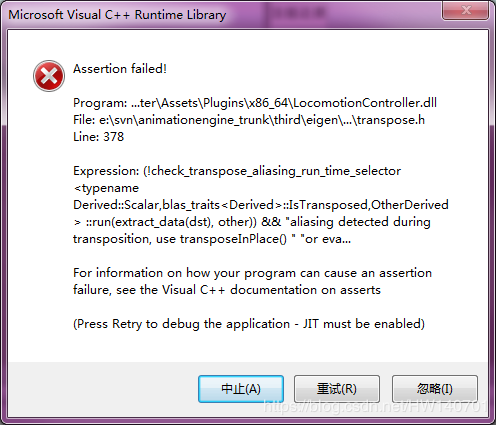int a= 10;
a=a/10;



Eigen::MatrixXd A= B * C;
A = A.transpose();Eigen::MatrixXd A;
Eigen::MatrixXd Acopy= B * C;
A = Acopy.transpose();


Eigen::MatrixXd A= B * C;
A = A.transposeInPlace();支付宝扫一扫微信扫一扫纵使晴明无雨色，入云深处亦沾衣。